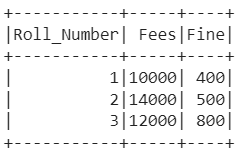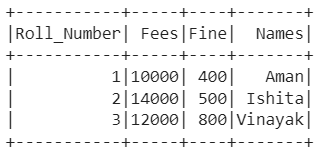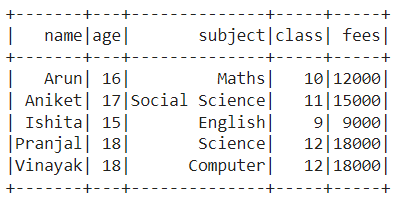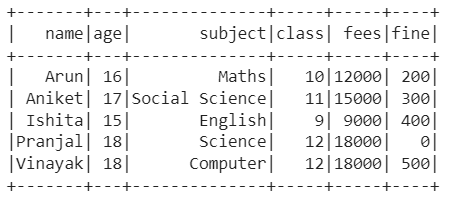GFG App
Open AppBrowser
Continue

# PySpark – Adding a Column from a list of values using a UDF

A data frame that is similar to a relational table in Spark SQL, and can be created using various functions in SparkSession is known as a Pyspark data frame. There occur various circumstances in which you get data in the list format but you need it in the form of a column in the data frame. If a similar situation has occurred with you, then you can do it easily by assigning increasing IDs to the data frame and then adding the values in a column. Read the article further to know more about it in detail.

### PySpark – Adding a Column from a list of values using a UDF

#### Example 1:

In the example, we have created a data frame with three columns ‘Roll_Number‘, ‘Fees‘, and ‘Fine‘ as follows:Once created, we assigned continuously increasing IDs to the data frame using the monotonically_increasing_id function. Also, we defined a list of values, i.e., student_names which need to be added as a column to a data frame. Then, with the UDF on increasing Id’s, we assigned values of the list as a column to the data frame and finally displayed the data frame after dropping the increasing Id’s column.

## Python3

 `# PySpark - Adding a Column from a ` `# list of values using a UDF ` ` `  `# Import the libraries SparkSession, ` `# functions, IntegerType, ` `# StringType, row_number, ` `# monotonically_increasing_id and Window ` `from` `pyspark.sql ``import` `SparkSession, functions as F ` `from` `pyspark.sql.types ``import` `IntegerType, StringType ` `from` `pyspark.sql.functions ``import` `row_number, monotonically_increasing_id ` `from` `pyspark.sql.window ``import` `Window ` ` `  `# Create a spark session using getOrCreate() function ` `spark_session ``=` `SparkSession.builder.getOrCreate() ` ` `  `# Create a user defined function to assign student names ` `# according to the row index by subtracting row number from 1 ` `labels_udf ``=` `F.udf(``lambda` `indx: student_names[indx``-``1``], StringType()) ` ` `  `# Create a data frame with three columns 'Roll_Number,' 'Fees' and 'Fine' ` `df ``=` `spark_session.createDataFrame( ` `    ``[(``1``, ``10000``, ``400``), (``2``, ``14000``, ``500``), (``3``, ``12000``, ``800``)],  ` `  ``[``'Roll_Number'``, ``'Fees'``, ``'Fine'``]) ` ` `  `# Define a list of elements ` `student_names ``=` `[``'Aman'``, ``'Ishita'``, ``'Vinayak'``] ` ` `  `# Create a column with continuous increasing Id's ` `df ``=` `df.withColumn(``"num_id"``, row_number().over( ` `    ``Window.orderBy(monotonically_increasing_id()))) ` ` `  `# Create a new column by calling the user defined function ` `new_df ``=` `df.withColumn(``'Names'``, labels_udf(``'num_id'``)) ` ` `  `# Delete the increasing Id's column and display the data frame ` `new_df.drop(``'num_id'``).show() `

Output:### Example 2:

In this example, we have used a data set (link), i.e., basically, a 5*5 data set as follows:Then, we assigned continuously increasing IDs to the data frame using the monotonically_increasing_id function. Also, we defined a list of values, i.e., fine_data which needs to be added as a column to the data frame. Then, with the UDF on increasing Id’s, we assigned values of the list as a column to the data frame and finally displayed the data frame after dropping the increasing Id’s column.

## Python3

 `# PySpark - Adding a Column from a list of values using a UDF ` ` `  `# Import the libraries SparkSession, functions, IntegerType, ` `# StringType, row_number, monotonically_increasing_id and Window ` `from` `pyspark.sql ``import` `SparkSession, functions as F ` `from` `pyspark.sql.types ``import` `IntegerType, StringType ` `from` `pyspark.sql.functions ``import` `row_number, monotonically_increasing_id ` `from` `pyspark.sql.window ``import` `Window ` ` `  `# Create a spark session using getOrCreate() function ` `spark_session ``=` `SparkSession.builder.getOrCreate() ` ` `  `# Create a user defined function to assign student names ` `# according to the row index by subtracting row number from 1 ` `labels_udf ``=` `F.udf(``lambda` `indx: fine_data[indx``-``1``], IntegerType()) ` ` `  `# Read the CSV file ` `df ``=` `csv_file ``=` `spark_session.read.csv( ` `    ``'/content/student_data.csv'``, sep``=``','``, inferSchema``=``True``, header``=``True``) ` ` `  `# Define a list of elements ` `fine_data ``=` `[``200``, ``300``, ``400``, ``0``, ``500``] ` ` `  `# Create a column with continuous increasing Id's ` `df ``=` `df.withColumn(``"num_id"``, row_number().over( ` `    ``Window.orderBy(monotonically_increasing_id()))) ` ` `  `# Create a new column by calling the user defined function ` `new_df ``=` `df.withColumn(``'fine'``, labels_udf(``'num_id'``)) ` ` `  `# Delete the increasing Id's column and display the data frame ` `new_df.drop(``'num_id'``).show() `

Output:My Personal Notes arrow_drop_up